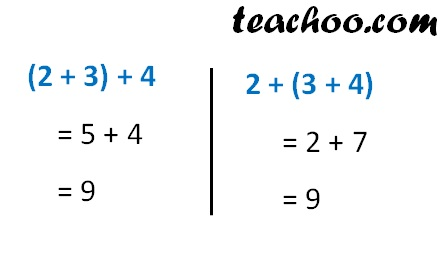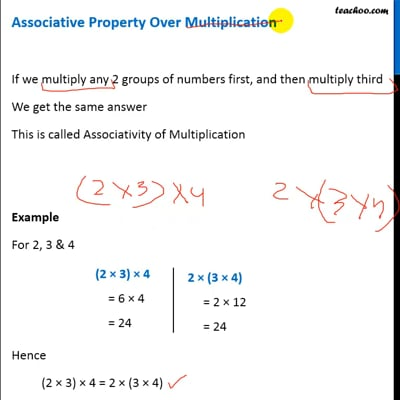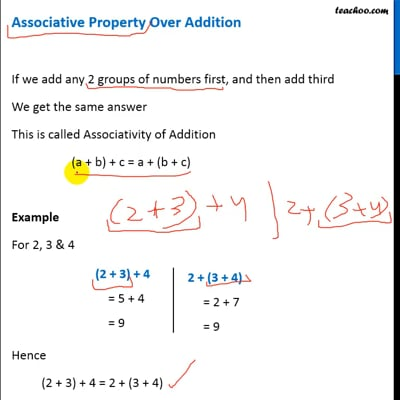Properties of whole numbers

Chapter 2 Class 6 Whole Numbers
Concept wise

If we add any 2 groups of numbers first, and then add third

This is called Associativity of Addition

(a + b) + c = a + (b + c)

Example

For 2, 3 & 4Hence

(2 + 3) + 4 = 2 + (3 + 4)This video is only available for Teachoo black usersThis video is only available for Teachoo black users

### Transcript

Associative Property Over Multiplication If we multiply any 2 groups of numbers first, and then multiply third We get the same answer This is called Associativity of Multiplication (a × b) × c = a × (b × c) Example For 2, 3 & 4 Hence (2 × 3) × 4 = 2 × (3 × 4)